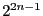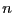Next: The SpectralRadiusConsistency procedure Up: Linear algebra Previous: Regularity of parametric matrices   Contents

### The RohnConsistency procedure

This procedure creates a matrix simplification program to help testing the regularity of a set of matrices. Its syntax is:
```
RohnConsistency(name)
```
where name indicates the name of the procedure, that will be written in the file name.C. This simplification procedure uses Rohn regularity test that is based on the calculation ofdeterminants of scalar matrices (whereis the dimension of the matrices). It shall therefore not be used with very large matrices.

Note that if the flag Use_Simp_Cond is set to 0 this test will not be used if the C++ flag Simp_In_Cond is not equal to 0. For example in RegularMatrix this is the case if the matrix received by Rohn is a conditioned matrix.

A remembering mechanism may allow to reduce the computation time by storing information on already processed matrix in the Rohn test. For using this mechanism you must set `ALIAS/rohn_remember` to the number of matrix that will be stored and `ALIAS/rohn_size_matrix` to the dimension of the matrix.Next: The SpectralRadiusConsistency procedure Up: Linear algebra Previous: Regularity of parametric matrices   Contents
Jean-Pierre Merlet 2012-12-20